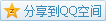# MIT OCW

## 18.02 Multivariable Calculus

### Double Integrals

#### Geometry and Definition

Like a regular integral, the double integral $\iint_{R}f(x,y)\mathrm{d}A$ represents the volume under the surface on the region $R$ where $\mathrm{d}A$ is a small piece of the region.
So we can have a summation like the following $$\iint_{R}f(x,y)\mathrm{d}A=\lim_{\Delta A_i\to 0}\sum f(x,y)\Delta A_i.$$

#### Convertion to Double "Integral"

In pratice, we will not calculate double integral by definition, and now we try to convert it to two single integrals.
The idea is to divide the volume into several slices along $x$ direction, and the volume of these slices are changing with $x$, so the slice determined by $x$ should be $$S(x)=\int_{y_{\min}(x)}^{y_{\max}(x)}f(x,y)\mathrm{d}y$$, and now we activate $x$, so the double integral becomes \begin{aligned}\iint_{R}f(x,y)\mathrm{d}A&=\int_{x_{\min}}^{x_{\max}}S(x)\mathrm{d}x\\&=\int_{x_{\min}}^{x_{\max}}\int_{y_{\min}(x)}^{y_{\max}(x)}f(x,y)\mathrm{d}y\mathrm{d}x.\end{aligned}
e.g. Calculate the integral of $z=1-x^2-y^2$ on the region $0\leq x\leq 1,0\leq y\leq 1$.
Solution \begin{aligned}\iint_{R}1-x^2-y^2\mathrm{d}y\mathrm{d}x&=\int_0^1\int_0^1 1-x^2-y^2\mathrm{d}y\mathrm{d}x\\&=\int_0^1\left[y-x^2y-\frac13y^3\right]^1_0\mathrm{d}x\\&=\int_0^1\frac23-x^2\mathrm{d}x\\&=\left[\frac23x-\frac13x^3\right]^1_0\\&=\frac13.\end{aligned}
e.g. Calculate the volume of $z=1-x^2-y^2$ above the $xy$ plane.
Solution Here the region is actually $x^2+y^2\leq 1$, and according to symmetric graph, we only need to calculate the quarter of the region and multiply $4$.
\begin{aligned}\iint_{R}1-x^2-y^2\mathrm{d}y\mathrm{d}x&=4\int_0^1\int_0^{\sqrt{1-x^2}} 1-x^2-y^2\mathrm{d}y\mathrm{d}x\\&=4\int_0^1\left[y-x^2y-\frac13y^3\right]^{\sqrt{1-x^2}}_0\mathrm{d}x\\&=4\int_0^1(1-x^2)\sqrt{1-x^2}-\frac13(1-x^2)\sqrt{1-x^2}\mathrm{d}x\\&=4\int_0^1\frac23(1-x^2)\sqrt{1-x^2}\mathrm{d}x\\(x=\sin(\theta))&=\frac83\int_{\frac\pi2}^0\cos^4(\theta)\mathrm{d}\theta\\&=\frac\pi2.\end{aligned}

#### Swap Orders

Last time we converted the volume into slices along $x$ direction, why not the other direction? Actually, it's feasible. And in theory, these two directions both work. The form along the other direction can be written as \begin{aligned}\iint_{R}f(x,y)\mathrm{d}A&=\int_{y_{\min}}^{y_{\max}}T(x)\mathrm{d}x\\&=\int_{y_{\min}}^{y_{\max}}\int_{x_{\min}(y)}^{x_{\max}(y)}f(x,y)\mathrm{d}x\mathrm{d}y.\end{aligned}
e.g. Evaluate $\int_0^1\int_{x}^{\sqrt{x}}\frac{e^y}{y}\mathrm{d}y\mathrm{d}x$.
Solution The region to be integrated is like the following.Here the expression is to make $y$ dependent on $x$, and now we swap the order and make $x$ dependent on $y$ that is \begin{aligned}\int_0^1\int_{x}^{\sqrt{x}}\frac{e^y}{y}\mathrm{d}y\mathrm{d}x&=\int_0^1\int_{y^2}^{y}\frac{e^y}{y}\mathrm{d}x\mathrm{d}y\\&=\int_0^1\left[\frac{e^y}{y}x\right]^{y}_{y^2}\mathrm{d}y\\&=\int_0^1 e^y(1-y)\mathrm{d}y\\&=\left[2e^y-ye^y\right]^1_0\\&=e-2.\end{aligned}

#### Polar Coordinates

In fact, sometimes it becomes easier when dealing the same problem in polar coordinates. The idea is to express $\mathrm{d}A$ in polar coordinates like the following picture.From the picture, it can be seen that the small piece of region becomes $$\mathrm{d}A=r\mathrm{d}r\mathrm{d}\theta.$$
e.g. Calculate the volume of $z=1-x^2-y^2$ above the $xy$ plane.
Solution The expression can be converted to $z=1-r^2$ and the region becomes $R:r\leq 1$, so the integral becomes \begin{aligned}\iint_{R}1-r^2\mathrm{d}A&=\int_0^{2\pi}\int_0^1(1-r^2)r\mathrm{d}r\mathrm{d}\theta\\&=\int_0^{2\pi}\left[\frac{r^2}{2}-\frac{r^4}{4}\right]^1_0\mathrm{d}\theta\\&=\int_0^{2\pi}\frac14\mathrm{d}\theta\\&=\left[\frac14\theta\right]^{2\pi}_0\\&=\frac\pi2.\end{aligned}

#### Application

• Get the area of a region on the plane
Given a region $R$ on the plane, we'd like to get its area. It's equivalent to evaluate $\iint_{R}\mathrm{d}A$, where $\mathrm{d}A$ depends on the type.
Variant Get the total mass of a flat object with the density $\delta$ function: $\iint_{r}\delta\mathrm{d}A$.
• Average
Like the single integral, an average of the function $f$ on a region $R$ can be defined as $$\bar{f}=\frac1{S_R}\iint_{R}f\mathrm{d}A,$$ where $S_R$ is the area of the region $R$.
Variant Weighted average: $$\tilde{f}=\frac1{M_R}\iint_{R}f\cdot\delta\mathrm{d}A,$$ where $M_R=\iint_{R}\delta\mathrm{d}A$.
Variant Center of mass of a flat object (in Cartesian plane): $$\bar{x}=\frac1{M_R}\iint_{R}x\cdot\delta\mathrm{d}A,\bar{y}=\frac1{M_R}\iint_{R}y\cdot\delta\mathrm{d}A,$$ where $M_R=\iint_{R}\delta\mathrm{d}A$.
• Moment of inertia
Given a point-like mass, the moment of inertia about some axis is defined $$I=mr^2,$$ where $m$ is the mass and $r$ is the distance to the axis.
Analogy The kinetic energy is $E_k=\frac12mv^2$, when we are push the mass, the kinetic energy will increase. So the larger $m$ is, the more difficult it will be to change the motion of the object, namely $v$. Similarly when the mass is rotating with angular velocity $\omega$, the velocity becomes $v=\omega\cdot r$, where $r$ is the distance to the axis and we get $E_k=\frac12mr^2\omega^2$, so $I=mr^2$ is also an indicator of the difficulty changing the rotation, namely $\omega$.

For a flat object, the moment of inertia becomes $$I=\iint_{R}r^2\cdot\delta\mathrm{d}A$$.
e.g. Compare the moment of inertia of a disk with radius $a$ about the center and about the point on the edge.
Solution When it's about the center, it looks like the following.Assume $\delta=1$, so just integrate it with polar way
\begin{aligned}I_0&=\iint_{R}r^2\mathrm{d}A\\&=\int_0^{2\pi}\int_0^a r^3\mathrm{d}r\mathrm{d}\theta\\&=\frac\pi2a^4.\end{aligned}
When it's about the edge point, we can change the origin.And do the same work
\begin{aligned}I_1&=\iint_{R}r^2\mathrm{d}A\\&=\int_0^{2\pi}\int_0^{2a\cos(\theta)} r^3\mathrm{d}r\mathrm{d}\theta\\&=\frac32\pi a^4.\end{aligned}
So $I_1=3I_0$.
Extension Parallel axis theorem